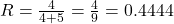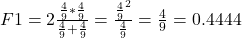###### A guide on regression error metrics (MSE, RMSE, MAE, MAPE, sMAPE, MPE) with Python code
2022-06-18###### Understanding Micro, Macro, and Weighted Averages for Scikit-Learn metrics in multi-class classification with example
2022-06-19

In one of my projects, I was wondering why I get the exact same value for precisionrecall, and the F1 score when using scikit-learn’s metrics. The project is about a multilabel classification problem where the input could be mapped to several classes. I was using micro averaging for the metric functions, which means the following according to sklearn’s documentation:

Calculate metrics globally by counting the total true positives, false negatives, and false positives.

According to the documentation, this behavior is correct:

Note that for “micro”-averaging in a multiclass setting with all labels included will produce equal precision, recall and F, while “weighted” averaging may produce an F-score that is not between precision and recall.

After thinking about it a bit I figured out why this is the case. In this post, I will explain the reasons.

## Definitions of precision, recall, and F1 score

First I will repeat the definitions of precision, recall, and the F1 score. Remember that True Positive samples (TP) are samples that were classified positive and are really positive. False Positive samples (FP) are samples that were classified positive but should have been classified negative. Analogously, False Negative samples (FN) were classified as negative but should be positive. Here, TP, FP, and FN stand for the respective number of samples in each of the classes.

Precision:Precision can be intuitively understood as the classifier’s ability to only predict really positive samples as positive. For example, a classifier that classifies just everything as positive would have a precision of 0.5 in a balanced test set (50% positive, 50% negative). One that has no false positives i.e. classifies only the true positives as positive would have a precision of 1.0. So basically, the fewer false positives a classifier gives, the higher its precision is.

RecallRecall can be interpreted as the number of positive test samples that were actually classified as positive. A classifier that just outputs positive for every sample, regardless if it is really positive, would get a recall of 1.0 but lower precision. The fewer false negatives a classifier gives, the higher is its recall.

So the higher precision and recall are, the better the classifier performs because it detects most of the positive samples (high recall) and does not detect many samples that should not be detected (high precision). In order to quantify that, we can use another metric called F1 score.

F1 score:This is just the weighted average between precision and recall. The higher precision and recall are, the higher the F1 score is. You can directly see from this formula, that if P=R, then F1=P=R, because:So this already explains why the F1 score is the same as precision and recall if precision and recall are the same. But why are recall and precision the same when using micro averaging? Let’s look at an example to understand this.

## Example: How to calculate precision, recall, and F1 score using micro averaging

In order to calculate precision and recall, we need to know the amount of TP, FP, and FN samples. How can you determine TP, FP, and FN when you have a non-binary problem, i.e. more than just positive and negative as output? Imagine you have 3 classes (1,2,3) and each sample belongs to exactly one class. The following table shows the predictions of our classifier for 9 test samples together with their correct labels.

TP is the number of samples predicted to have the correct label. In this example, TP = 4 (all green cells)
FP is the number of labels that got a “vote” but shouldn’t. For example, in the first column, 1 should have been predicted, but 2 was predicted. So there is a false positive for class 2 in this case. On the other hand, if the prediction is right (column 2), there is no FP counted. In this example, FP = 5 (all red cells)
FN is the number of labels that should have been predicted but weren’t. Look at the first column again. 1 should have been predicted, but wasn’t. So there is an FN for class 1 in this case. As in the FP case, there is no FN counted if the prediction is correct (column 2). In this example, FP = 5 (all red cells)

In other words, if there is a false positive, there will always also be a false negative and vice versa, because always one class is predicted. If class A is predicted and the true label is B, then there is an FP for A and an FN for B. If the prediction is correct, i.e. class A is predicted and A is also the true label, then there is neither a false positive nor a false negative but only a true positive. So there is no possibility that would increase only FP or FN but not both. That is why precision and recall are always the same when using the micro averaging scheme.

Now let’s actually calculate the values of precision, recall, and F1 score.

Precision:Recall:F1 score:We can see that all metric values are identical.

Note: Since micro averaging does not distinguish between different classes and then just averages their metric scores, this averaging scheme is not prone to inaccurate values due to an unequally distributed test set (e.g. 3 classes and one of these has 98% of the samples). This is why I prefer this scheme over the macro averaging scheme. Besides micro averaging, one might also consider weighted averaging in the case of an unequally distributed data set.

## Macro averaging and weighted averaging

Note that the explanation above is only true when using micro averaging! When using macro averaging, the implementation is working as follows (source: sklearn documentation):

Calculate metrics for each label, and find their unweighted mean. This does not take label imbalance into account.

In this case, for each class 1,2,3 the values for precision, recall, and F1 score are calculated separately and then averaged regardless of their occurrence ratio in the dataset. So if two classes only occur 1% each and the third class occurs 98% and the bigger class is always predicted correctly but the smaller often wrong, then the F1 score would still be very bad while it would be good with micro averaging or weighted averaging.

When using weighted averaging, the occurrence ratio would also be considered in the calculation, so in that case, the F1 score would be very high (as only 2% of the samples are predicted mainly wrong). It always depends on your use case and what you should choose. If the smaller classes are very important, then probably the weighted approach would be a bad choice and you should go for macro averaging.

## Example: Macro averaging

For the sake of completeness, I am also going to show how precision, recall and F1 score are calculated when using macro averaging instead of micro averaging. In this case, we first have to look at each class separately. Now we can treat every class as a binary label (class predicted yes/no).

In the previous example (see table above), each class has the following TP, FN, FP values and the following precision (P), recall (R) and F1 scores:

Class 1: TP = 0 / FN = 2 / FP = 2 => P = 0 / R = 0 / F1 = 0
Class 2: TP = 3 / FN = 1 / FP = 2 => P = 35 / R = 34 / F1 = 23
Class 3: TP = 1 / FN = 2 / FP = 1 => P = 12 / R = 13 / F1 = 25

All classes combined:
TP = 4 / FN = 5 / FP = 5 (by the way, these are the same values as in the micro average example!)

Precision (average over all classes): 0.36667
Recall (average over all classes): 0.36111
F1 (average over all classes): 0.35556

These values differ from the micro averaging values! They are much lower than the micro averaging values because class 1 has not even one true positive, so very bad precision and recall for that class.

The scores obtained using weighted average would be closer to the micro-average scores as this also respects class imbalances [just an intuitive guess that I have not proved formally yet].

I am skipping a full example of the weighted averaging scheme, but the only difference would be that instead of weighting every class by 1, you would weight it by the number of samples in your test data and then divide the sum by the number of samples in all classes together.

## Code example for micro, macro, and weighted averaging

In case you are wondering how to use the metrics with scikit-learn (sklearn) with the different averages, here is some Python 3 code for you:

from sklearn.metrics import precision_score, recall_score, f1_score

# These values are the same as in the table above
labels      = [1,2,3,2,3,3,1,2,2]
predicitons = [2,2,1,2,1,3,2,3,2]

print("Precision (micro): %f" % precision_score(labels, predicitons, average='micro'))
print("Recall (micro):    %f" % recall_score(labels, predicitons, average='micro'))
print("F1 score (micro):  %f" % f1_score(labels, predicitons, average='micro'), end='\n\n')

print("Precision (macro): %f" % precision_score(labels, predicitons, average='macro'))
print("Recall (macro):    %f" % recall_score(labels, predicitons, average='macro'))
print("F1 score (macro):  %f" % f1_score(labels, predicitons, average='macro'), end='\n\n')

print("Precision (weighted): %f" % precision_score(labels, predicitons, average='weighted'))
print("Recall (weighted):    %f" % recall_score(labels, predicitons, average='weighted'))
print("F1 score (weighted):  %f" % f1_score(labels, predicitons, average='weighted'))


Output:

Precision (micro): 0.444444
Recall (micro): 0.444444
F1 score (micro): 0.444444

Precision (macro): 0.366667
Recall (macro): 0.361111
F1 score (macro): 0.355556

Precision (weighted): 0.433333
Recall (weighted): 0.444444
F1 score (weighted): 0.429630


Resource:

https://simonhessner.de/why-are-precision-recall-and-f1-score-equal-when-using-micro-averaging-in-a-multi-class-problem/##### Amir Masoud Sefidian
Machine Learning Engineer

#### 1 Comment

1. […] A more detailed explanation of this observation could be found in this post. […]